Related Articles

# Mathematics | Set Operations (Set theory)

• Difficulty Level : Easy
• Last Updated : 05 Mar, 2021

### Union

Union of the sets A and B, denoted by A ∪ B, is the set of distinct elements that belong to set A or set B, or both.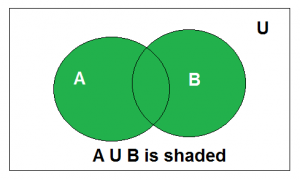Venn diagram of A ∪ B

Above is the Venn Diagram of A U B.

Example: Find the union of A = {2, 3, 4} and B = {3, 4, 5};
Solution : A ∪ B = {2, 3, 4, 5}.

### Intersection

The intersection of the sets A and B, denoted by A ∩ B, is the set of elements that belong to both A and B i.e. set of the common elements in A and B.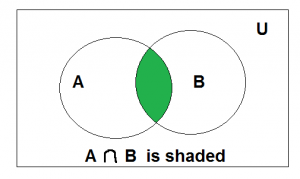Venn diagram of A ∩ B

Above is the Venn Diagram of A ∩ B.

Example: Find the intersection of A = {2, 3, 4} and B = {3, 4, 5}
Solution : A ∩ B = {3, 4}.

### Disjoint

Two sets are said to be disjoint if their intersection is the empty set. i.e, sets have no common elements.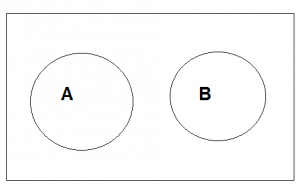Above is the Venn Diagram of A disjoint B.

Example: Let A = {1, 3, 5, 7, 9} and B = { 2, 4, 6, 8}
A and B are disjoint sets since both of them have no common elements.

### Set Difference

The difference between sets is denoted by ‘A – B’, which is the set containing elements that are in A but not in B. i.e., all elements of A except the element of B.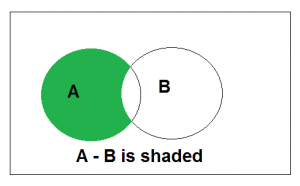Above is the Venn Diagram of A-B.

Example: If A = {1, 2, 3, 4, 5} and B = { 2, 4, 6, 8}, find A-B
Solution: A-B = {1, 3, 5}

### Complement

The complement of a set A, denoted by AC is the set of all the elements except the elements in A. Complement of the set A is U – A.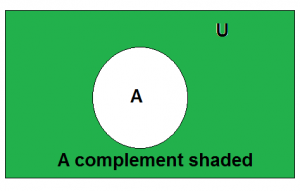Above is the Venn Diagram of Ac

Example: Let U = {1, 2, 3, 4, 5, 6, 7, 8, 9, 10} and A = {2, 4, 6, 8}.
Find AC
Solution: AC = U-A = {1, 3, 5, 7, 9, 10}

Addition of sets A and B, referred to as Minkowski addition, is the set in whose elements are the sum of each possible pair of elements from the 2 sets (that is one element is from set A and the other is from set B).
Set subtraction follows the same rule, but with the subtraction operation on the elements. It is to be observed that these operations are operable only on numeric data types. Even if operated otherwise, it would only be a symbolic representation without any significance. Further, it can be seen easily that set addition is commutative, while subtraction is not.[Tex]A-B=A\cap \bar{B}  [/Tex]

1. Associative Properties: A ∪ (B ∪ C) = (A ∪ B) ∪ C and A ∩ (B ∩ C) = (A ∩ B) ∩ C
2. Commutative Properties: A ∪ B = B ∪ A and A ∩ B = B ∩ A
3. Identity Property for Union: A ∪ φ = A
4. Intersection Property of the Empty Set: A ∩ φ = φ
5. Distributive Properties: A ∪ (B ∩ C) = (A ∪ B) ∩ (A ∪ C) similarly for intersection.

Attention reader! Don’t stop learning now.  Practice GATE exam well before the actual exam with the subject-wise and overall quizzes available in GATE Test Series Course.

Learn all GATE CS concepts with Free Live Classes on our youtube channel.

My Personal Notes arrow_drop_up